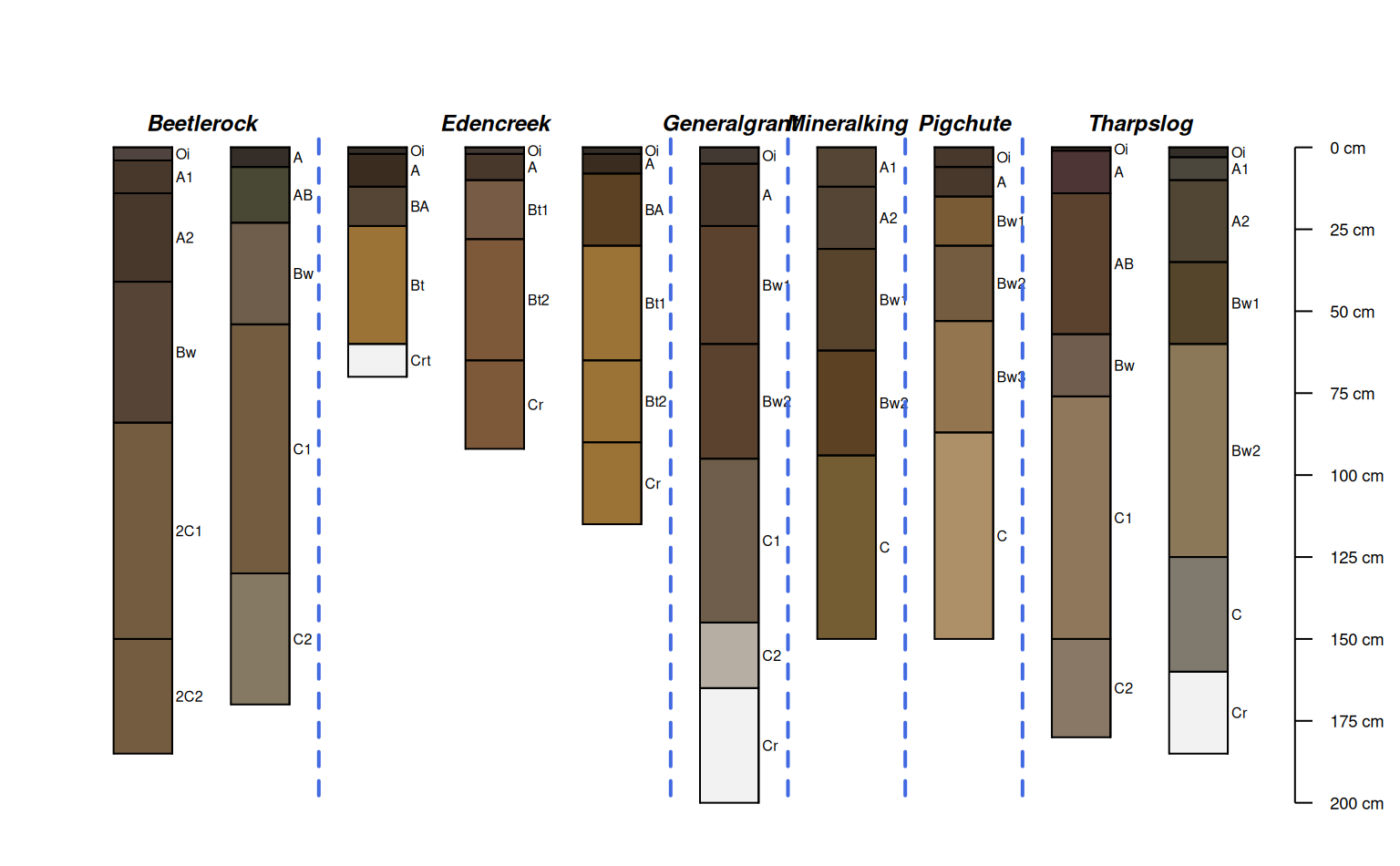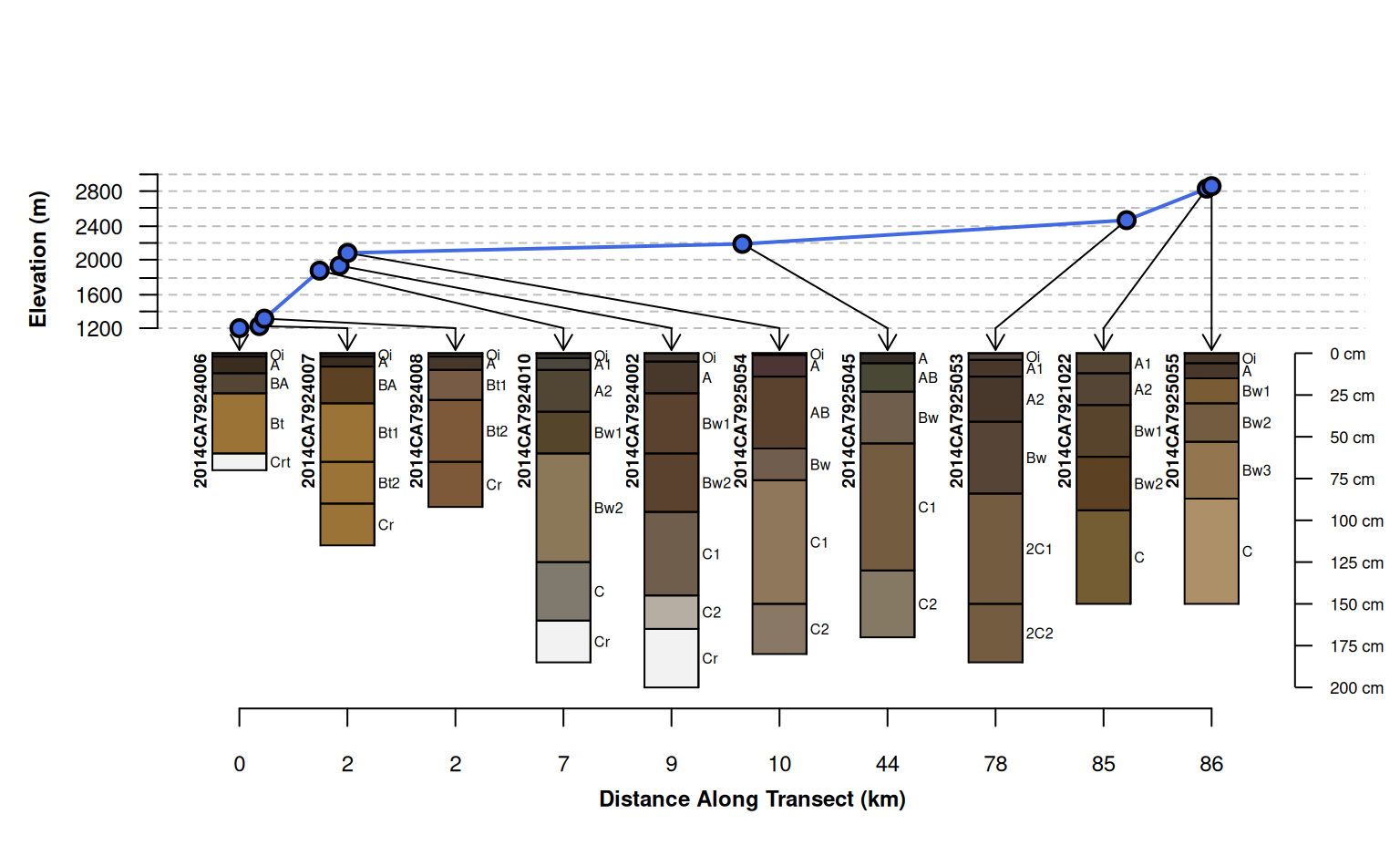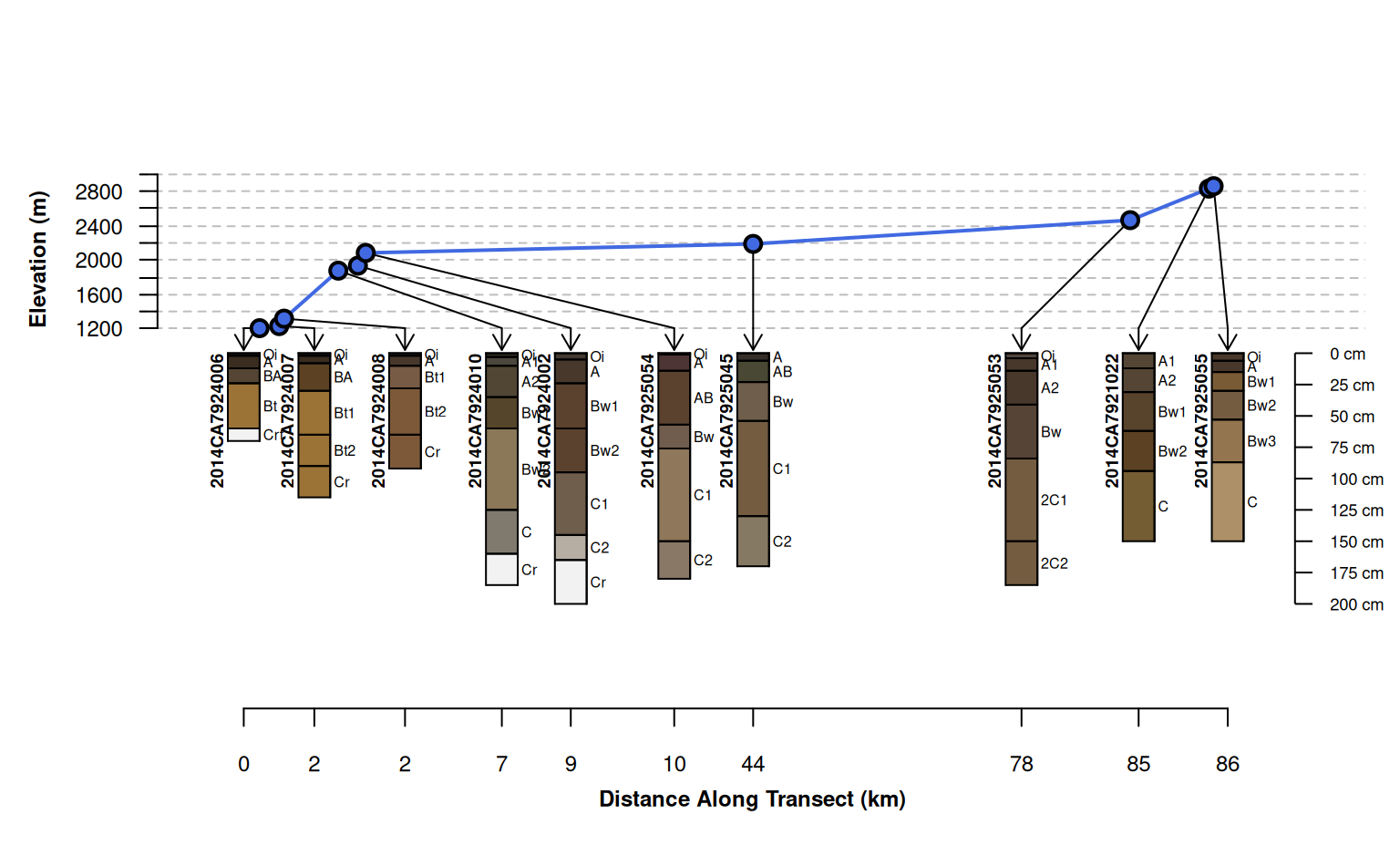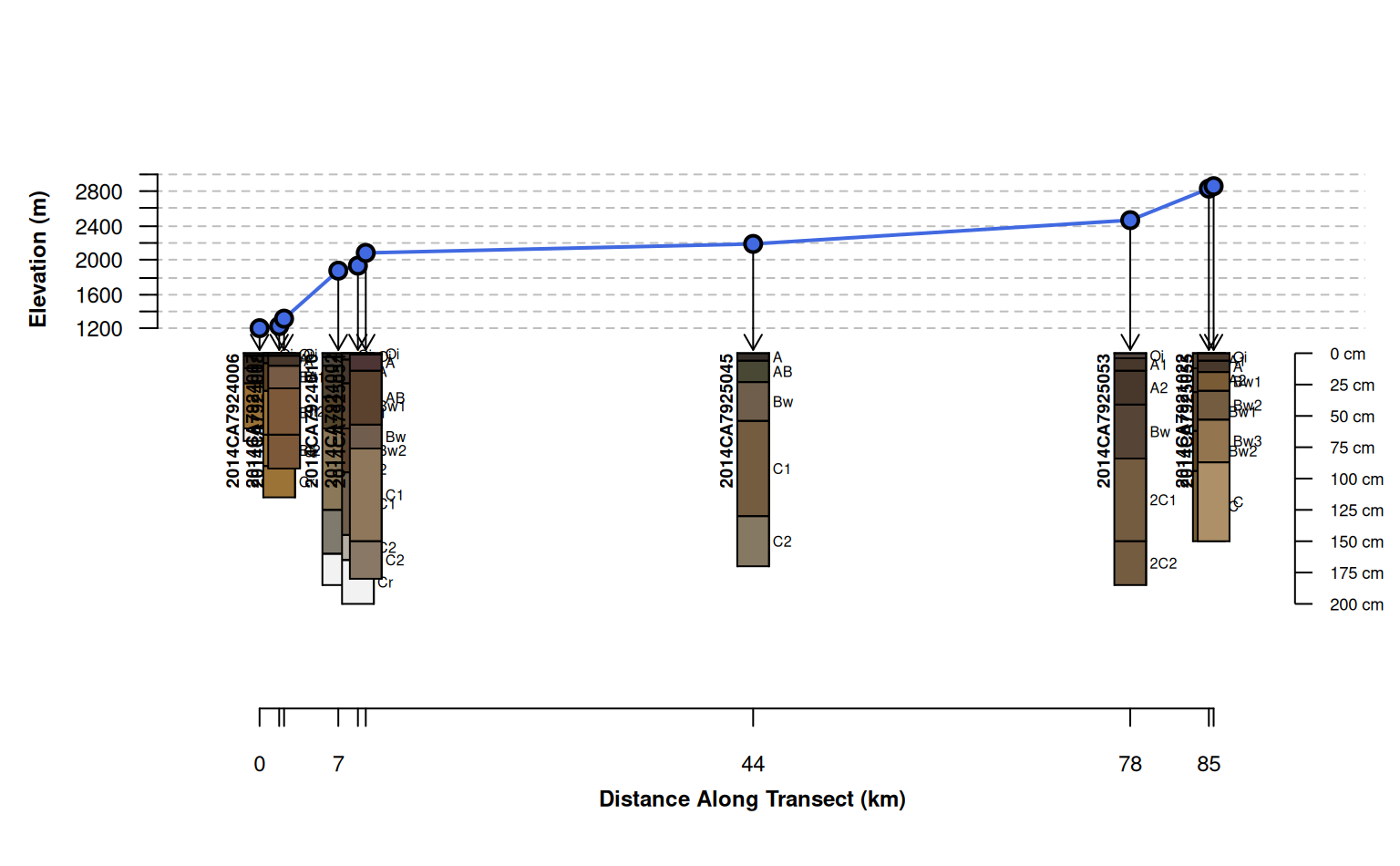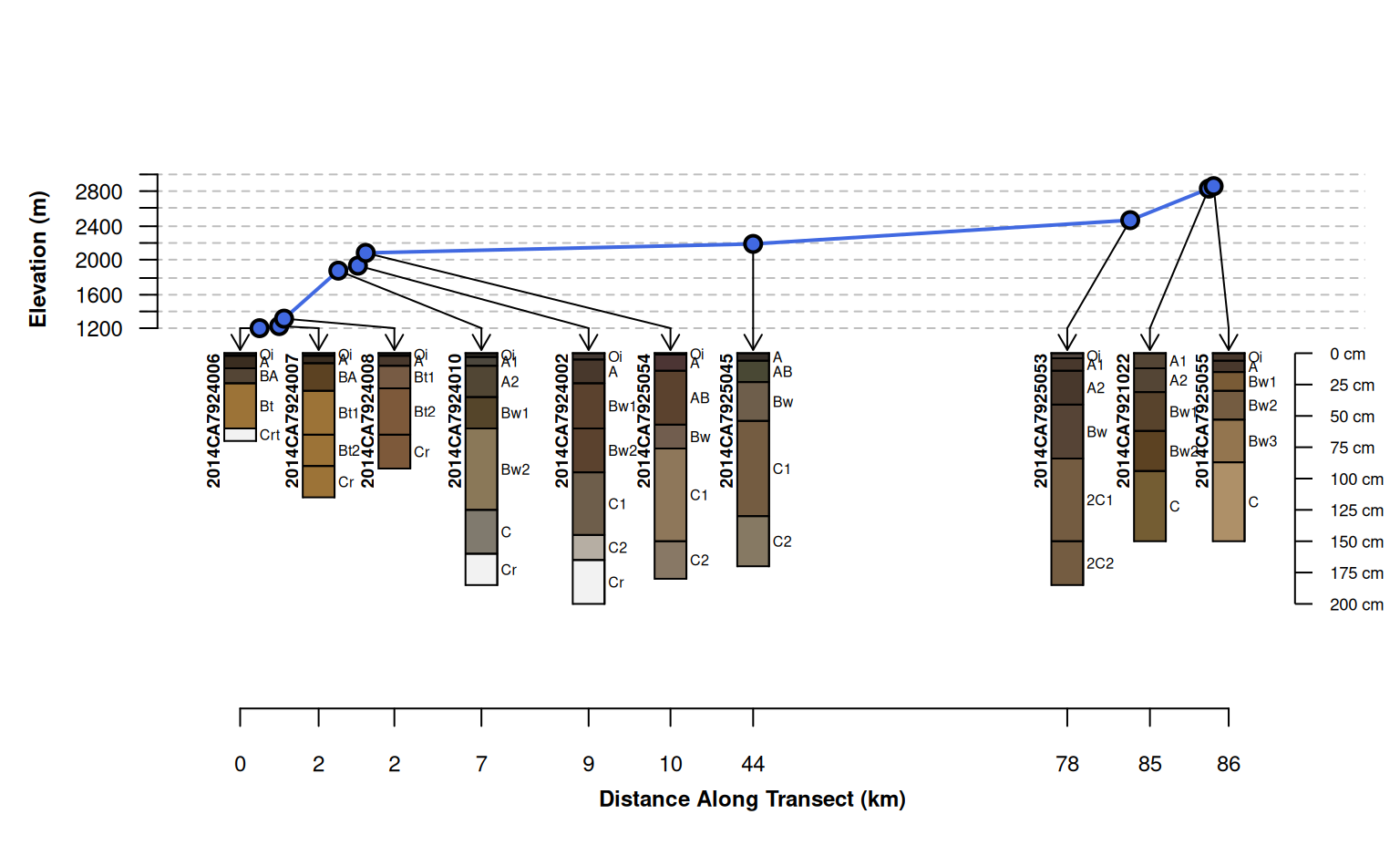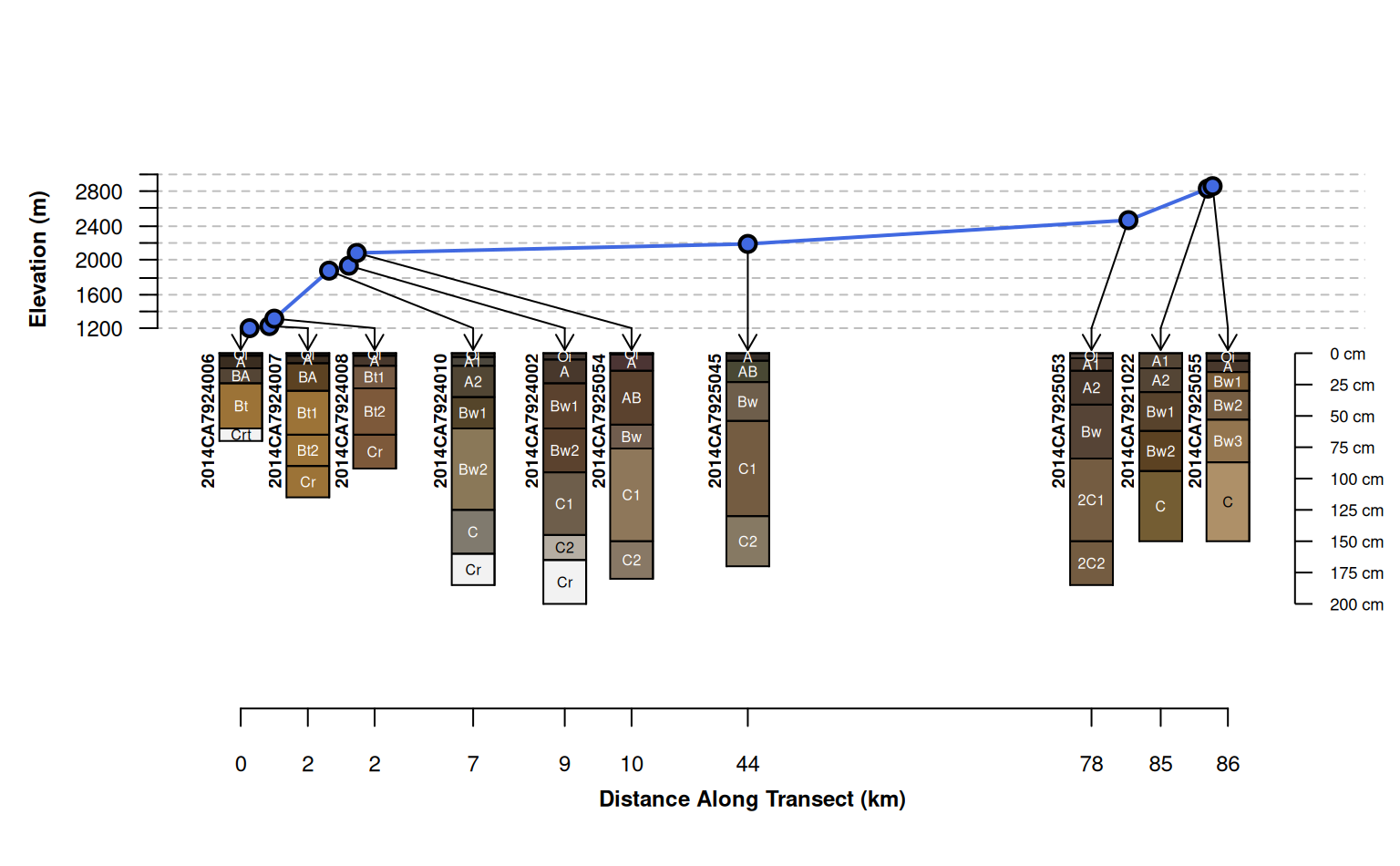Plot a collection of Soil Profiles linked to their position along some gradient (e.g. transect).

plotTransect(
s,
transect.col = "RoyalBlue",
tick.number = 7,
y.offset = 100,
scaling.factor = 0.5,
distance.axis.title = "Distance Along Transect (km)",
crs = NULL,
dist.scaling.factor = 1000,
spacing = c("regular", "relative"),
fix.relative.pos = list(thresh = 0.6, maxIter = 5000),
...
)

## Arguments

s

SoilProfileCollection object

the name of a site-level attribute containing gradient values

optional indexing vector used to override sorting along grad.var.name

transect.col

color used to plot gradient (transect) values

tick.number

number of desired ticks and labels on the gradient axis

y.offset

vertical offset used to position profile sketches

scaling.factor

scaling factor applied to profile sketches

distance.axis.title

a title for the along-transect distances

crs

an optional CRS object (sp package) used to convert coordinates into a projected coordinate reference system

a title for the gradient axis

dist.scaling.factor

scaling factor (divisor) applied to linear distance units, default is conversion from m to km (1000)

spacing

profile sketch spacing style: "regular" (profiles aligned to an integer grid) or "relative" (relative distance along transect)

fix.relative.pos

adjust relative positions in the presence of overlap, FALSE to suppress, otherwise list of arguments to aqp::fixOverlap

...

further arguments passed to aqp::plotSPC.

## Value

An invisibly-returned data.frame object:

• scaled.distance: cumulative distance, scaled to the interval of 0.5, nrow(coords) + 0.5

• distance: cumulative distance computed along gradient, e.g. transect distance

• x: x coordinates, ordered by gradient values

• y: y coordinate, ordered by gradient values

• grad.order: a vector index describing the sort order defined by gradient values

## Details

Depending on the nature of your SoilProfileCollection and associated gradient values, it may be necessary to tinker with figure margins, y.offset and scaling.factor.

## Note

This function is very much a work in progress, ideas welcome!

D.E. Beaudette

## Examples


# \donttest{

if(require(aqp) &
require(sp) &
require(soilDB)
) {

# sample data
data("mineralKing", package = "soilDB")

# device options are modified locally, reset when done

# quick overview
par(mar=c(1,1,2,1))
groupedProfilePlot(mineralKing, groups='taxonname', print.id=FALSE)

# init coords and CRS
coordinates(mineralKing) <- ~ x_std + y_std

# projected CRS, units of meters

par(mar=c(4.5,4,4,1))

# standard transect plot, profile sketches arranged along integer sequence

# default behavior, attempt adjustments to prevent over-plot and preserve relative spacing
# use set.seed() to fix outcome
name='hzname', width=0.15, spacing = 'relative')

# attempt relative positioning based on scaled distances, no corrections for overlap
# profiles are clustered in space and therefore over-plot
width=0.15, spacing = 'relative', fix.relative.pos = FALSE)

# customize arguments to aqp::fixOverlap()
width=0.15, spacing = 'relative',

width=0.2, spacing = 'relative',
name.style = 'center-center')

par(op)

}